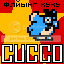-=+=- -=+=- -=+=- -=+=- -=+=- -=+=- -=+=- -=+=- -=+=- -=+=- -=+=- -=+=- -=+=- -=+=- -=+=- -=+=- -=+=- -=+=- -=+=- -=+=- -=+=- -=+=- -=+=- -=+=- -=+=- -=+=- -=+=- -=+=- -=+=- -=+=- (c) WidthPadding Industries 1987 0|37|0 -=+=- -=+=- -=+=- -=+=- -=+=- -=+=- -=+=- -=+=- -=+=- -=+=- -=+=- -=+=- -=+=- -=+=- -=+=- -=+=- -=+=- -=+=- -=+=- -=+=- -=+=- -=+=- -=+=- -=+=- -=+=- -=+=- -=+=- -=+=- -=+=- -=+=- SoCoder -> Link Home -> Models/Textures For all your 3D related links. Older -->Free 3D characters! Yay! Afr0 Artist-3D Tons of free models! Erebel55Roadkill UV Tool flying_cucco12 Places for free Stock Photos SchererererFree Game Arts Free game art directory cthugGreat 3D renders (SG1) Jayenkai50 Free Rusted Metal Textures Title says it all SchererererDexsoft free barrels and crates model pack HoboBenLoopix Project models+textures HoboBenSoftimage|XSI Mod Tool free 3d modelling & animation tool svrmanPsionic's 3D Game Resources Site full of 2D/3D tutorials, tonnes of free 3d game models, textures and SFX, active forums, etc. HoboBenDarkroom Studios Model Packs Affordable, proffessional model packs for 3D programs HoboBenAnim8or Free, easy to use 3D modeller HoboBenNOCTUA Graphics Amazing free textures! HoboBenTurbo Squid free textures Wowie HoboBenMisfit Model 3D Another 3D modeller - this one looks great! HoboBenQuake 2 Modeller Vertice by vertice and faces modelling HoboBenBiturn converter Converts 3D file formats HoboBen Older -->